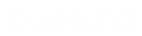Feedback

• Contents

# Comparing Datetime Values

The date comparison functions for years and months return a value that represents the difference in dates only for the relevant component of the date.  For example, in performing #DiffYearsTZ or #DiffYearsUTC, it is the actual year that is being looked at, not the number of 365 day periods that have elapsed between the two dates. So, for example, if #DiffYearsTZ is performed on the dates December 30th, 1997 and January 2nd, 1998, it would return a value of 1 even though the two dates are only three days apart.

The date comparison functions for days, hours, minutes, and seconds, however, convert each date to a numeric value and then return a value that represents the date difference in a number of units of seconds (60 seconds for DiffMinutes, 3,600 seconds for DiffHours, and 86,400 seconds for DiffDays).

## DiffYearsTZ

DiffYearsTZ(DateTime1, DateTime2, TZ)

Returns an integer to indicate the year difference between DateTime1 and DateTime2, as expressed in the timezone TZ.

The "year difference" can be thought of as the absolute value of:

GetYearTZ(DateTime1, TZ) – GetYearTZ(DateTime2, TZ)

If there is an error calculating the difference, -1 is returned.

## DiffYearsUTC

DiffYearsUTC( DateTime1, DateTime2 )

Returns an integer to indicate the year difference between DateTime1 and DateTime2, as expressed in UTC.

The "year difference" can be thought of as the absolute value of:

GetYearUTC(DateTime1) – GetYearUTC(DateTime2)

If there is an error calculating the difference, -1 is returned.

## DiffMonthsTZ

DiffMonthsTZ(DateTime1, DateTime2, TZ)

Returns an integer to indicate the month difference between DateTime1 and DateTime2, as expressed in the TZ timezone.

The "month difference" can be thought of as the absolute value of:

GetYearTZ(DateTime1, TZ)*12

+GetMonthTZ(DateTime1, TZ)) – (GetYearTZ(DateTime2, TZ)*12

+GetMonthTZ(DateTime2,TZ))

If there is an error calculating the difference, -1 is returned.

## DiffMonthsUTC

DiffMonthsUTC( DateTime1, DateTime2 )

Returns an integer to indicate the month difference between DateTime1 and DateTime2, as expressed in UTC.

The "month difference" can be thought of as the absolute value of:

(GetYearUTC(DateTime1)*12

+GetMonthUTC(DateTime1)) – (GetYearUTC(DateTime2)*12

+GetMonthUTC(DateTime2))

If there is an error calculating the difference, -1 is returned.

## DiffDays

DiffDays (DateTime1, DateTime2)

Returns an integer to indicate the number of 86,400-second units between the two DateTime values. (86,400 = 24 hours x 60 minutes x 60 seconds)

## DiffHours

DiffHours (DateTime1, DateTime2)

Returns an integer to indicate the number of 3,600-second units between the two DateTime values. (3,600 equals 60 minutes x 60 seconds.)

## DiffMinutes

DiffMinutes (DateTime1, DateTime2)

Returns an integer to indicate the number of 60-second units between the two DateTime values.

## DiffSeconds

DiffSeconds (DateTime1, DateTime2)

Returns an integer to indicate the number of seconds between the two DateTime values.

Related Topics

Date/Time Functions - Using Time Zones versus Universal Coordinated time (UTC)